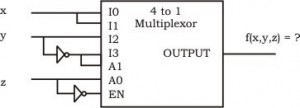71. A device employing INTR line for device interrupt puts the CALL instruction on the data bus while a. INTA is active b. HOLD is active c. READY is active d. None of these

 72. In 8085, which of the following modifies the program counter ? a. Only PCHL instruction b. Only ADD instructions c. Only JMP and CALL instructions d. All instructions

 73. The 2' s complement representation of the decimal value - 15 is a. 1111 b. 11111 c. 111111 d. 10001

 74. Sign extension is a step in a. floating point multiplication b. signed 16 bit integer addition c. arithmetic left shift d. converting a signed integer from one size to another
 Answer: (d).converting a signed integer from one size to another

 75. In 2' s complement addition, overflow a. is flagged whenever there is carry from sign bit addition b. cannot occur when a positive value is added to a negative value c. is flagged when the carries from sign bit and previous bit match d. none of the above
 Answer: (b).cannot occur when a positive value is added to a negative value

 76. In the absolute addressing mode a. the operand is inside the instruction b. the address of the operand is inside the instruction c. the register containing address of the operand is specified inside the instruction d. the location of the operand is implicit

 77. Consider the following multiplexer where 10, 11, 12, 13 are four data input lines selected by two address line combinations A1A0 = 00, 01, 10, 11 respectively and f is the output of the multiplexer. EN is the enable input. The function f(x, y, z) implemented by the circuit is :a. xyz' b. xy + z c. x + z d. None of these